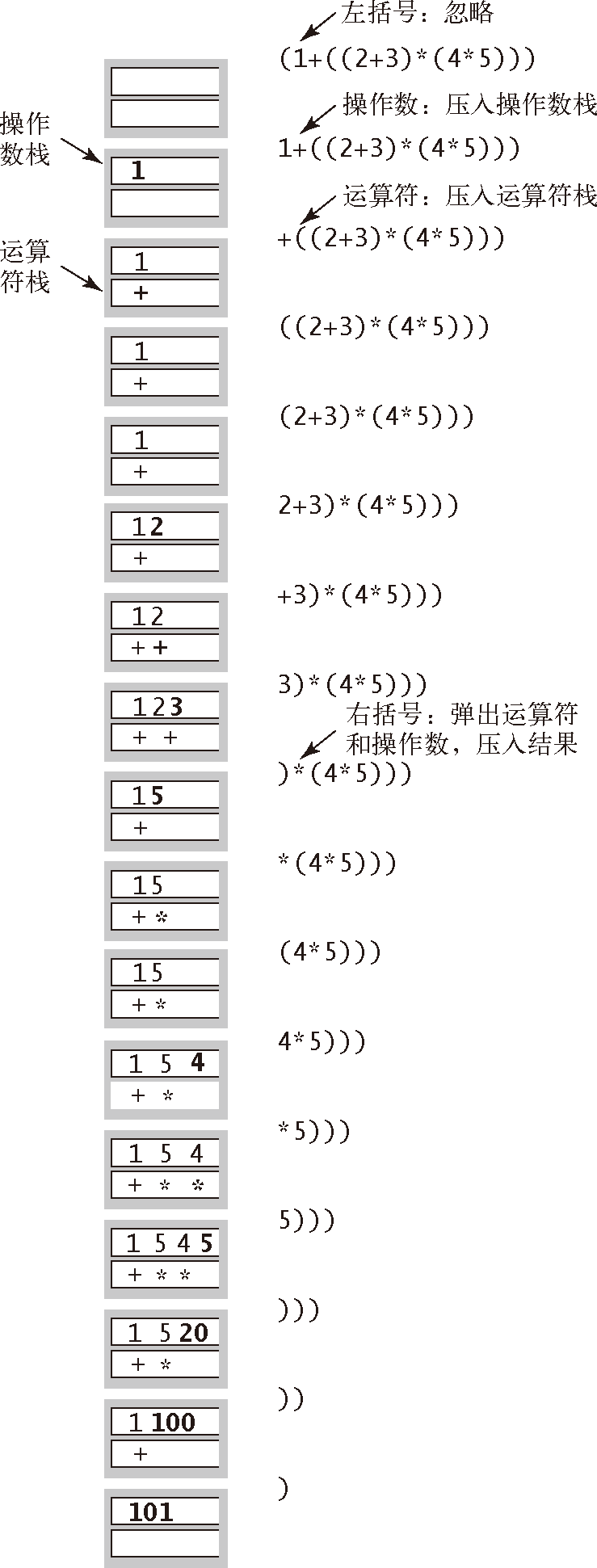# 算法（4th ed）(122)：基础——背包、队列和栈 5.1.7(API：算术表达式求值）

( 1 + ( ( 2 + 3 ) * ( 4 * 5 ) ) )• 操作数压入操作数栈；
• 运算符压入运算符栈；
• 忽略括号；
• 在遇到括号时，弹出一个运算符，弹出所需数量的操作数，并将运算符和操作数的运算结果压入操作数栈。

( 1 + ( ( 2 + 3 ) * ( 4 * 5 ) ) )( 1 + ( 5 * ( 4 * 5 ) ) )( 1 + ( 5 * 20 ) )( 1 + 100 )101

Dijkstra 的双栈算术表达式求值算法

public class Evaluate{  public static void main(String[] args)  {     Stack<String> ops  = new Stack<String>();     Stack<Double> vals = new Stack<Double>();     while (!StdIn.isEmpty())     {  // 读取字符，如果是运算符则压入栈        String s = StdIn.readString();        if      (s.equals("("))               ;        else if (s.equals("+"))    ops.push(s);        else if (s.equals("-"))    ops.push(s);        else if (s.equals("*"))    ops.push(s);        else if (s.equals("/"))    ops.push(s);        else if (s.equals("sqrt")) ops.push(s);        else if (s.equals(")"))        {  // 如果字符为 ")"，弹出运算符和操作数，计算结果并压入栈           String op = ops.pop();           double v = vals.pop();           if      (op.equals("+"))    v = vals.pop() + v;           else if (op.equals("-"))    v = vals.pop() - v;           else if (op.equals("*"))    v = vals.pop() * v;           else if (op.equals("/"))    v = vals.pop() / v;           else if (op.equals("sqrt")) v = Math.sqrt(v);           vals.push(v);        }  // 如果字符既非运算符也不是括号，将它作为 double 值压入栈        else vals.push(Double.parseDouble(s));     }     StdOut.println(vals.pop());  }}

% java Evaluate( 1 + ( ( 2 + 3 ) * ( 4 * 5 ) ) )101.0 % java Evaluate( ( 1 + sqrt ( 5.0 ) ) / 2.0 )1.618033988749895